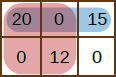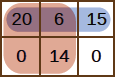## Game

Bazza and Shazza are playing a game. The board is a grid of cells, with R rows numbered 0, …, R - 1, and C columns, numbered 0, …, C - 1. We let (P, Q) denote the cell in row P and column Q. Each cell contains a non-negative integer, and at the beginning of the game all of these integers are zero.

The game proceeds as follows. At any time, Bazza may either:

• update a cell (P, Q), by assigning the integer that it contains
• ask Shazza to calculate the greatest common divisor (GCD) of all integers within a rectangular block of cells, with opposite corners (P, Q) and (U, V) inclusive.

Bazza will take NU + NQ actions (updating cells NU times and asking questions NQ times) before he gets bored and goes outside to play cricket.

### Example

Suppose R = 2 and C = 3, and Bazza begins with the following updates:

• Update cell (0, 0) to 20;
• Update cell (0, 2) to 15;
• Update cell (1, 1) to 12.The resulting grid is shown in the picture above. Bazza might then ask for GCDs in the following rectangles:

• Opposite corners (0, 0) and (0, 2): The three integers in this rectangle are 20, 0 and 15, and their GCD is 5.
• Opposite corners (0, 0) and (1, 1): The four integers in this rectangle are 20, 0, 0 and 12, and their GCD is 4.

Now suppose Bazza makes the following updates:

• Update cell (0, 1) to 6;
• Update cell (1, 1) to 14.The new grid is shown in the picture above. Bazza might then ask for GCDs in the following rectangles again:

• Opposite corners (0, 0) and (0, 2): Now the three integers in this rectangle are 20, 6 and 15, and their GCD is 1.
• Opposite corners (0, 0) and (1, 1): Now the four integers in this rectangle are 20, 6, 0 and 14, and their GCD is 2.

Here Bazza has performed NU = 5 updates and NQ = 4 questions.

Given the size of the grid, you should implement the operations `update` and `calculate` as described below.

To help you, below is a function `gcd2(X, Y)` to compute the greatest common divisor of two non-negative integers X and Y. If X = Y = 0 then `gcd2(X, Y)` will return 0 also. This function is fast enough to score full points; in particular, the running time is at worst proportional to log(X + Y).

C/C++Pascal
``````long long gcd2(long long X, long long Y) {
long long tmp;
while (X != Y && Y != 0) {
tmp = X;
X = Y;
Y = tmp % Y;
}
return X;
}``````
``````function gcd2(X, Y : Int64) : Int64;
var
tmp : Int64;
begin
while (X <> Y) and (Y > 0) do begin
tmp := X;
X := Y;
Y := tmp mod Y;
end;
gcd2 := X;
end;``````

You will first be given the initial size of the grid, allowing you to initialise any variables and data structures.

Parameters:

• R: The number of rows (1 ≤ R ≤ 109).
• C: The number of columns (1 ≤ C ≤ 109).

`update`: This operation will be called when Bazza assigns the number in some grid cell.

Parameters:

• P: The row of the grid cell (0 ≤ PR - 1).
• Q: The column of the grid cell (0 ≤ QC - 1).
• K: The new integer in this grid cell (0 ≤ K ≤ 1018). May be the same as the current value.

`calculate`: This operation should calculate the greatest common divisor of all integers in the rectangle with opposite corners (P, Q) and (U, V). This range is inclusive, i.e., the cells (P, Q) and (U, V) are included in the rectangle.

If all integers in this rectangle are zero, then this function should return zero also.

Parameters:

• P: The row of the top-left cell in the rectangle (0 ≤ PR - 1).
• Q: The column of the top-left cell in the rectangle (0 ≤ QC - 1).
• U: The row of the bottom-right cell in the rectangle (0 ≤ UR - 1).
• V: The column of the bottom-right cell in the rectangle (0 ≤ VC - 1).
• When this operation is called, you should output the GCD of all integers in the rectangle, or 0 if all of those integers are zero.

### Input Format

The input will be in the following format:

• line 1: `R C N`
• next N lines: one action per line, in the order in which actions occur

The line for each action must be in one of the following formats:

• To indicate `update`: `1 P Q K`
• To indicate `calculate`: `2 P Q U V`

### Output Format

For every call of `calculate`, output an integer on a single line - the GCD of all integers in the calculated rectangle, or 0 if all of those integers are zero.

### Sample Input and Description

The following case describes the example above.

InputDescriptionOutput
`2 3 9`
`R = 2, C = 3, N = 9`
`1 0 0 20``update 0 0 20`
`1 0 2 15``update 0 2 15`
`1 1 1 12``update 1 1 12`
`2 0 0 0 2``calculate 0 0 0 2``5`
`2 0 0 1 1``calculate 0 0 1 1``4`
`1 0 1 6``update 0 1 6`
`1 1 1 14``update 1 1 4`
`2 0 0 0 2``calculate 0 0 0 2``1`
`2 0 0 1 1``calculate 0 0 1 1``2`

110≤ 100≤ 100≤ 100≤ 100
227≤ 10≤ 100,000≤ 10,000≤ 250,000
326≤ 2,000≤ 2,000≤ 10,000≤ 250,000
417≤ 109≤ 109≤ 10,000≤ 250,000
520≤ 109≤ 109≤ 22,000≤ 250,000

Point Value: 30 (partial)
Time Limit: 16.00s
Memory Limit: 512M

Languages Allowed:
C++03, PAS, C, HASK, ASM, RUBY, PYTH2, JAVA, PHP, SCM, CAML, PERL, C#, C++11, PYTH3

• (5/0)
Sorry Daniel, but upon inspection, I found that your solution doesn't actually have the correct worst-case complexity, so I added a counter-example case for extra fun :)

• (7/0)
Nope, I'm afraid that's definitely not a legit optimization - added another case :D

• (3/0)
You'd better add one more, then :D. I passed with a quad tree + speedups using properties of GCD + a different quad tree approach for your cases.

• (0/1)
http://wcipeg.com/submissions/status/392189

I keep getting SIGABRT, am I using too much memory or is it something else?

• (0/0)
If you're getting SIGABRT then an exception is being thrown.

If you're not throwing an exception, the STL must be.

If you're not using checked vector access, then the exception is probably bad_alloc.

• (0/0)
http://wcipeg.com/submissions/status/386558

On test 3d i'm getting RE,however on my computer i get AC on first 5 tests of subtask 2,what's happening?

• (0/0)
You're getting SIGABRTs, which probably indicates you're exceeding the memory limit on an STL allocation. I checked your submission and confirmed this is the case.

This information is listed in the README.

• (14/1)
Can you introduce a short circuit on batch cases so as soon as one test case fails, the rest of the batch does not run? There is really no point in running every single case if a solution does not work since its only partial points by batch.

• (3/0)
it's alot easier to solve this offlinely, at IOI we can't do that, still stuck at 80 at doing this onlinely :(

• (4/0)
One submission takes 8 minutes to grade...

• (4/0)
There's not much I can do about this. Once I get a full-time job I'll consider moving the Judge to a dedicated server with lots of cores, which might alleviate the problem...